ChemTalk

# Intro to Energy## Core Concepts

In this article, you will learn about what energy is. You will also learn about work, heat transfer, and endothermic + exothermic reactions. Finally you’ll learn about kinetic and potential energy, and a formula for each.

## What is Energy?

Energy is the capacity to supply heat or do work.

Work is the energy that gets transferred when something is moved or pushed against an opposing force. A common example used to illustrate work in chemistry is a gas trapped inside a cylinder with a piston on top. If the gas molecules start to move around more and hit the piston harder, they’re applying a force to the piston. If this force is strong enough, it can make the piston move upward. This is the gas doing work – it’s using its energy to move the piston. The formula for work is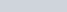, where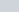is work in Joules,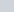is force in Newtons, and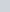is displacement in meters. Learn more about work and how to calculate it here.

In chemical reactions, work can happen when substances change in certain ways. For example, if a gas expands during a reaction and pushes against a piston, it’s doing work, because the molecules of the gas are opposing the gravity force holding the piston down.An example of a chemical reaction which does work by pushing up on the piston its contained in. The opposing force here is gravity.

## But what is heat transfer?

Heat transfer is the transfer of energy between substances that are at different temperatures. Think of a cup of hot tea left on a table. Over time, the heat from the tea transfers to the cooler air in the room, causing the tea to gradually cool down and the air in the room to slightly heat up. This transfer occurs because the faster-moving particles in the hot tea collide with the slower-moving particles in the air, transferring their energy and warming the air around the cup. This natural flow of energy from warmer to cooler substances is what we call heat transfer.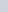represents heat in chemistry, not to be confused with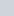, the reaction quotient. Specifically, it refers to the heat transferred during a process. A negative value ofmeans that a reaction is exothermic, or releases heat, and a positive value ofmeans that a reaction is endothermic, or takes in heat. A common mistake people learning about heat make is mixing these terms up. Remember that these terms are about the system, and not the surroundings. So while an endothermic reaction occurring in a flask, for example, will feel cold to the touch, this is because the reaction itself is taking in heat, making it endothermic. The opposite applies to exothermic reactions.The system is where the reaction takes place, and the surroundings are everything around the system

## Potential and Kinetic Energy

Kinetic energy is the energy of motion. It’s the energy an object possesses due to its movement. Think of a moving car – it has kinetic energy because it’s in motion. In chemistry, particles like atoms and molecules also have kinetic energy when they move. The formula for calculating kinetic energy (KE) in Joules is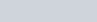, were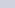is mass in kilograms and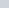is velocity in m/s.

Potential energy is a concept that describes stored energy within an object due to its position or condition. It’s like a “stored possibility” that can be converted into other forms of energy when conditions change. Imagine a ball on a high shelf – it has potential energy because it could fall and gain kinetic energy. This transformation of energy is another important concept in chemistry, and it’s referred to as the law of conservation of energy, or the first law of thermodynamics. It states that energy can never be created or destroyed, only transformed from one form to another.The balloon has potential energy stored in the compressed air within it. When you let go it will move upward because the higher-pressure air inside the balloon pushes against the lower-pressure air outside. This movement is an example of potential energy being converted into kinetic energy as the balloon rises.

## What types of potential energy are there?

Chemical potential energy is one of the most common types for chemists. When substances undergo chemical reactions, the rearrangement of atoms involves the conversion of bonds to energy. Batteries are also another example of chemical potential energy that we use in our daily lives. Some other notable types include nuclear, thermal, elastic, and gravitational.

Gravitational potential energy is simplest to calculate and what most people think of when they hear “potential energy”. In the previous example of the ball on the high shelf, the potential energy of the ball was in the form of gravitational potential energy, or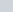. The formula to calculate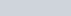, whereis mass in kg,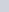is the acceleration due to gravity (roughly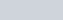, andis the height of the object in meters.is in Joules.

Additionally, similar to Joules, calories are another unit of energy which is often used, usually in the context of food. A calorie is defined as a energy equivalent to the heat energy needed to raise the temperature of 1 gram of water by 1 °C, or approximately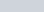.kcal is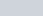cal.